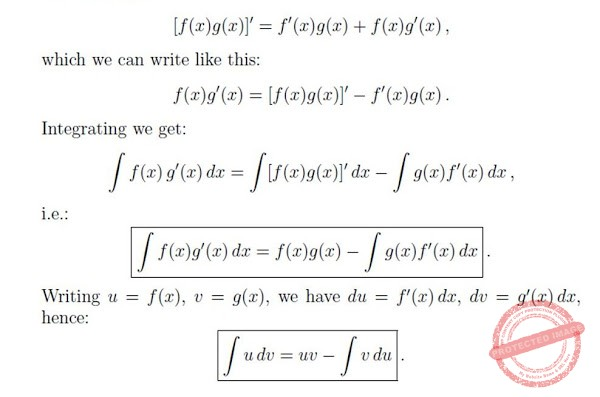# Lecture in Integration by Parts

(Last Updated On: December 21, 2017)

We learned the fact that the Integration is the inverse of Differentiation. For Every differentiation rule there is a corresponding integration rule. Like the Substitution Rule for integration corresponds to the Chain Rule for differentiation. Now, the rule that corresponds to the Product Rule for differentiation is called the rule for integration by parts.

### The Formula

The method of integration by parts is based on the product rule for differentiation:### The Steps

• The ability to choose u and dv correctly.
• If the choice is right, the new integral that  you obtain  is simpler than the original one.
• Integrate using the Integration by parts formula
• Check the answer by differentiating.

### The Examples

1.

Let:               u = x                           dv = ex dx
then:           du = dx                           v = e

Solution:

Check by differentiating:

2.

Let:               u = x                           dv = sin (x) dx
then:           du = dx                           v = -cos (x)

Solution:

P inoyBIX educates thousands of reviewers and students a day in preparation for their board examinations. Also provides professionals with materials for their lectures and practice exams. Help me go forward with the same spirit.

“Will you subscribe today via YOUTUBE?”

Subscribe

PinoyBIX Engineering. © 2014-2020 All Rights Reserved | How to Donate? |